## Excel WEIBULL Function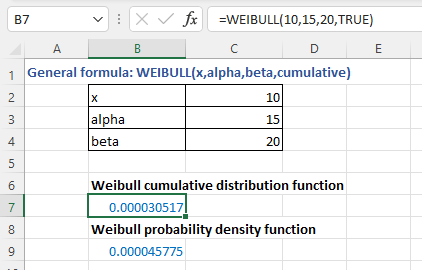### Description

The Excel WEIBULL function returns the Weibull probability density function or Weibull cumulative distribution for a supplied set of parameters. It is frequently used for reliability analysis, e.g., for testing the mean time of the failure of an equipment in a specific time range.

Note: This function has been replaced with one or more new functions that may provide improved accuracy after Excel 2010 version. This function is still can be available but considering it may be not available in the future, you should better study to use the new function from now.

Formula syntax

WEIBULL(x_range,alpha,betta,cumulative)

Arguments

 x: Required, the value at which to evaluate the function. alpha: Required, a parameter of the distribution. beta: Required, a parameter of the distribution. cumulative: Required, a logical value that determines which form of the function to be used. TRUE: Weibull cumulative distribution function is used;< FALSE: Weibull probability density function is used.

### Returns

WEIBULL function returns a numeric value.

### Remarks

1. The function returns #VALUE! error value if x, alpha, or beta is a non-numeric value.

2. The function returns #NUM! error value when

• x is negative;
• alpha or beta ≤ 0
• X_range and prob_range have different numbers of data points, WEIBULL function return the #N/A error value.

3. The equation for Weibull cumulative distribution function is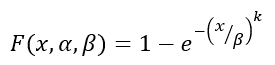4. The equation for Weibull probability density function is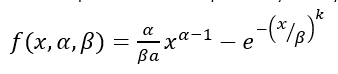5. When alpha = 1, WEIBULL function returns the exponential distribution with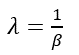### Version

Excel 2007 and earlier versions

### Usage and Examples

Basic usage

For getting the Weibull cumulative distribution function (cumulative as TURE) and Weibull probability density function (cumulative as FALSE) separately for the supplied x (10), alpha (15),beta (20), you can use the WEIBULL function as these:

=WEIBULL(10,15,20,TRUE) // Weibull cumulative distribution function

=WEIBULL(10,15,20,FALSE) // Weibull probability density function

Press Enter key to get the result.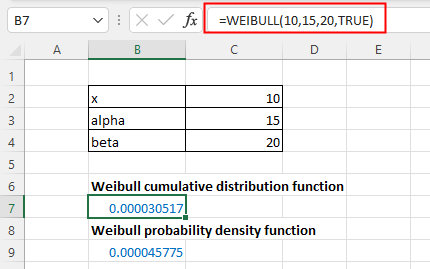#### Other Functions:

• Excel POISSON Function
The Excel POISSON function returns the Poisson distribution which is used to predict the number of events occurring over a specific time.

• Excel FDIST Function
The Excel FDIST Function returns the (right-tailed) F probability distribution which is usually used to measure the degree of diversity between two data sets.

• Excel EXPON.DIST Function
EXPON.DIST function used in Excel 2010, returns the exponential distribution based on the given x value and the parameter of the distribution.

• Excel F.DIST Function
The Excel F.DIST function returns the F probability distribution which is usually used to measure the degree of diversity between two data sets.

### The Best Office Productivity Tools

#### Kutools for Excel - Helps You To Stand Out From Crowd

 Popular Features: Find, Highlight or Identify Duplicates  |  Delete Blank Rows  |  Combine Columns or Cells without Losing Data  |  Round without Formula ... Super VLookup: Multiple Criteria  |  Multiple Value  |  Across Multi-Sheets  |  Fuzzy Lookup... Adv. Drop-down List: Easy Drop Down List  |  Dependent Drop Down List  |  Multi-select Drop Down List... Column Manager: Add a Specific Number of Columns  |  Move Columns  |  Toggle Visibility Status of Hidden Columns  |  Compare Columns to Select Same & Different Cells ... Featured Features: Grid Focus  |  Design View  |  Big Formula Bar  |  Workbook & Sheet Manager | Resource Library (Auto Text)  |  Date Picker  |  Combine Worksheets  |  Encrypt/Decrypt Cells  |  Send Emails by List  |  Super Filter  |  Special Filter (filter bold/italic/strikethrough...) ... Top 15 Toolsets:  12 Text Tools (Add Text, Remove Characters ...)  |  50+ Chart Types (Gantt Chart ...)  |  40+ Practical Formulas (Calculate age based on birthday ...)  |  19 Insertion Tools (Insert QR Code, Insert Picture from Path ...)  |  12 Conversion Tools (Numbers to Words, Currency Conversion ...)  |  7 Merge & Split Tools (Advanced Combine Rows, Split Excel Cells ...)  |  ... and more

Kutools for Excel Boasts Over 300 Features, Ensuring That What You Need is Just A Click Away...#### Office Tab - Enable Tabbed Reading and Editing in Microsoft Office (include Excel)

• One second to switch between dozens of open documents!
• Reduce hundreds of mouse clicks for you every day, say goodbye to mouse hand.
• Increases your productivity by 50% when viewing and editing multiple documents.
• Brings Efficient Tabs to Office (include Excel), Just Like Chrome, Edge and Firefox.